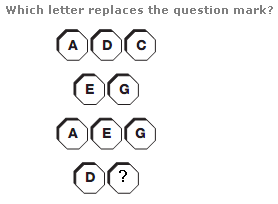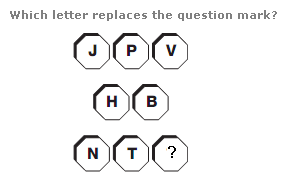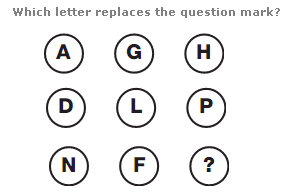# Puzzles - Missing letters puzzles

### Exercise :: Missing letters puzzlesAnswer : C Explanation : Convert each letter to it’s numerical value, and read horizontal lines as 2 or 3 digit numbers. Add the top 3 digit number to the 2 digit number below to give 200, repeat this pattern for the 3 and 2 digit numbers underneath.Answer : Z Explanation : Starting at the top left of the diagram, and moving around it in a Z shape, letters follow the alphabetic sequence, in steps of 6 letters at a time.Answer : T Explanation : Working in rows, the numerical value of the letter in the right hand column equals the sum of the numerical values of the letters in the left and central columns.